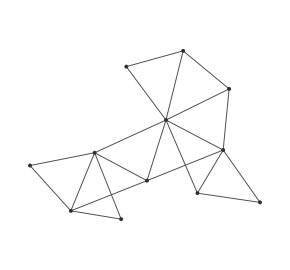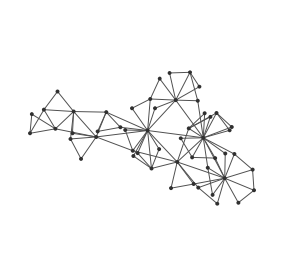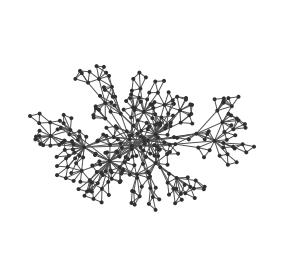# Dorogovtsev-Mendes graph generator

This generator creates graph using the Dorogovtsev and Mendes algorithm. This starts by creating three nodes and tree edges, making a triangle, and then add one node at a time. Each time a node is added, an edge is chosen randomly and the node is connected via two new edges to the two extremities of the chosen edge.

This process generates a power-low degree distribution, as nodes that have more edges have more chances to be selected since their edges are more represented in the edge set.

The Dorogovtsev - Mendes algorithm always produce planar graphs.

## Usage

The more this generator is iterated, the more nodes are generated. It can therefore generate trees of any size. A each call to `nextEvents()`, a new node and two edges are added.## Complexity

At each step only one node and two edges are added.

## Example

Here is how this generator can be used:

This kind of graph is described, among others, in the “Evolution of networks” by Dorogovtsev and Mendes.

• Evolution of networks,
• S. N. Dorogovtsev and J. F. F. Mendes,
• Adv. Phys. 51, pp. 1079 – 1187, 2002,
• arXiv:cond-mat/0106144v2